Subscribe to our newsletter to receive the latest news and events from TWI:

# Mechanical testing - Tensile testing, Part 1

Mechanical testing is carried out to produce data that may be used for design purposes or as part of a material joining procedure or operator acceptance scheme. The most important function may be that of providing design data since it is essential that the limiting values that a structure can withstand without failure are known.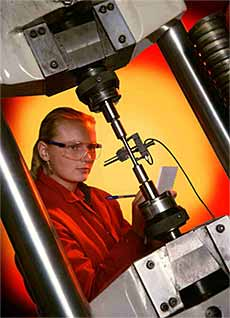Fig.1. Typical tensile testing machine

Inadequate control of the material properties by the supplier, or incompetent joining procedures and operatives are, however, equally crucial to the supply of a product that is safe in use. An example of this dual role of mechanical testing is the tensile test that may be used either to determine the yield strength of a steel for use in design calculations or to ensure that the steel complies with a material specification's strength requirements.

##Mechanical tests may also be divided into quantitative or qualitative tests. A quantitative test is one that provides data that will be used for design purposes, a qualitative test where the results will be used for making comparisons - hardness or Charpy-V tests - for example as a 'go/no go test' such as the bend test.

Mechanical property data are obtained from a relatively small number of standard tests and these will be covered over the next several articles. These will include tensile and toughness tests, the tests used for welding procedure and welder approval and those used for the determination of in-service properties.

## Tensile testing

As mentioned earlier the tensile test is used to provide information that will be used in design calculations or to demonstrate that a material complies with the requirements of the appropriate specification - it may therefore be either a quantitative or a qualitative test.

The test is made by gripping the ends of a suitably prepared standardised test piece in a tensile test machine and then applying a continually increasing uni-axial load until such time as failure occurs. Test pieces are standardised in order that results are reproducible and comparable as shown in Fig 2.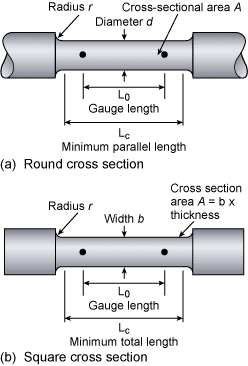Fig.2. Standard shape tensile specimens

Specimens are said to be proportional when the gauge length, L0, is related to the original cross sectional area, A0, expressed as L0 =k√A0 . The constant k is 5.65 in EN specifications and 5 in the ASME codes. These give gauge lengths of approximately 5x specimen diameter and 4x specimen diameter respectively - whilst this difference may not be technically significant it is important when claiming compliance with specifications.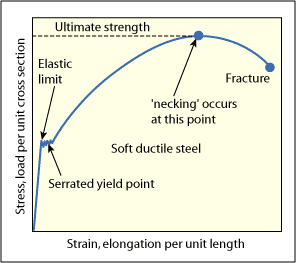Fig.3. Stress/strain curve

Both the load (stress) and the test piece extension (strain) are measured and from this data an engineering stress/strain curve is constructed, Fig.3. From this curve we can determine:

a) the tensile strength, also known as the ultimate tensile strength, the load at failure divided by the original cross sectional area where the ultimate tensile strength (U.T.S.), σmax = Pmax /A0 , where Pmax = maximum load, A0 = original cross sectional area. In EN specifications this parameter is also identified as 'Rm';

b) the yield point (YP), the stress at which deformation changes from elastic to plastic behaviour ie below the yield point unloading the specimen means that it returns to its original length, above the yield point permanent plastic deformation has occurred, YP or σy = Pyp /A0 where Pyp = load at the yield point. In EN specifications this parameter is also identified as 'Re ';

c) By reassembling the broken specimen we can also measure the percentage elongation, El% how much the test piece had stretched at failure where El% = (Lf - L0 /Lo ) x100 where Lf = gauge length at fracture and L0 = original gauge length. In EN specifications this parameter is also identified as 'A' ( Fig.4a).

d) the percentage reduction of area, how much the specimen has necked or reduced in diameter at the point of failure where R of A% =(A0 - Af /A0 ) x 100 where Af = cross sectional area at site of the fracture. In EN specifications this parameter is also identified as 'Z', ( Fig.4b).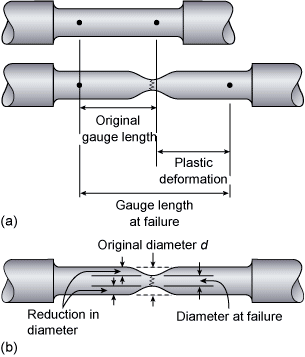Fig.4: a) Calculation of percentage elongation, b) Calculation of percentage reduction of area

(a) and (b) are measures of the strength of the material, (c) and (d) indicate the ductility or ability of the material to deform without fracture.

The slope of the elastic portion of the curve, essentially a straight line, will give Young's Modulus of Elasticity, a measure of how much a structure will elastically deform when loaded.

A low modulus means that a structure will be flexible, a high modulus a structure that will be stiff and inflexible.

To produce the most accurate stress/strain curve an extensometer should be attached to the specimen to measure the elongation of the gauge length. A less accurate method is to measure the movement of the cross-head of the tensile machine.

The stress strain curve in Fig.3 shows a material that has a well pronounced yield point but only annealed carbon steel exhibits this sort of behaviour. Metals that are strengthened by alloying, by heat treatment or by cold working do not have a pronounced yield and some other method must be found to determine the 'yield point'.

This is done by measuring the proof stress ( offset yield strength in American terminology), the stress required to produce a small specified amount of plastic deformation in the test piece.

The proof stress is measured by drawing a line parallel to the elastic portion of the stress/strain curve at a specified strain, this strain being a percentage of the original gauge length, hence 0.2% proof, 1% proof (see Fig.5).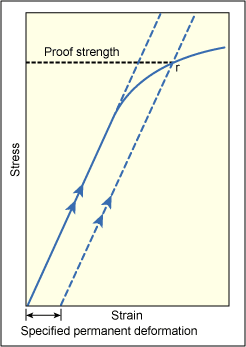Fig.5. Determination of proof (offset yield) strength

For example, 0.2% proof strength would be measured using 0.2mm of permanent deformation in a specimen with a gauge length of 100mm. Proof strength is therefore not a fixed material characteristic, such as the yield point, but will depend upon how much plastic deformation is specified. It is essential therefore when considering proof strengths that the percentage figure is always quoted. Most steel specifications use 0.2% deformation, RP0.2 in the EN specifications.

Some materials such as annealed copper, grey iron and plastics do not have a straight line elastic portion on the stress/strain curve. In this case the usual practice, analogous to the method of determining proof strength, is to define the 'yield strength' as the stress to produce a specified amount of permanent deformation.

Part 2 of this series on mechanical testing will cover welding procedure approval tensile testing.